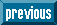## Indicators

•Cited by SciELO
•Access statistics

•Similars in SciELO

## Print version ISSN 1405-5546

#### Abstract

CLEMPNER, Julio; MEDEL, Jesús  and  CARSTEANU, Alin. Setting Decision Process Optimization into Stochastic vs. Petri Nets Contexts. Comp. y Sist. [online]. 2007, vol.10, n.3, pp.301-322. ISSN 1405-5546.

In this work we introduce a new modeling paradigm for developing decision process representation for shortest-path problem and games. Whereas in previous work, attention was restricted to tracking the net using as a utility function Bellman's equation, this work uses a Lyapunov-like function. In this sense, we are changing the traditional cost function by a trajectory-tracking function which is also an optimal cost-to-target function for tracking the net. This makes a significant difference in the conceptualization of the problem domain, allowing the replacement of the Nash equilibrium point by the Lyapunov equilibrium point in shortest-path game theory. Two different formal theoretic approaches are employed to represent the problem domain: i) Markov decision process and, ii) place-transitions Petri Nets having as a feature a Markov decision process, called Decision Process Petri nets (DPPN). The main point of this paper is its ability to represent the system-dynamic and trajectory-dynamic properties of a decision process. Within the system-dynamic properties framework we prove new notions of equilibrium and stability. In the trajectory-dynamic properties framework, we optimize the trajectory function value used for path planning via a Lyapunov-like function, obtaining as a result new characterizations for final decision points (optimum points) and stability. We show that the system-dynamic and Lyapunov trajectory-dynamic properties of equilibrium, stability and final decision points (optimum points) meet under certain restrictions. Moreover, we generalize the problem to join with game theory. We show that the Lyapunov equilibrium point coincides with the Nash equilibrium point under certain restrictions. As a consequence, all the properties of equilibrium and stability are preserved in game theory under certain restrictions. This is the most important contribution of this work. The potential of this approach remains in its formal proof simplicity for the existence of an equilibrium point. To the best of our knowledge the approach seems to be new in decision process, game theory and Petri Nets.

Keywords : shortest-path problem; shortest-path game; stability; Lyapunov; Markov decision process; Petri nets.

· abstract in Spanish     · text in Spanish     · Spanish (pdf )All the contents of this journal, except where otherwise noted, is licensed under a Creative Commons Attribution License